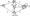# Finite Automata and Formal Languages December 2010

Note ; 1. Answer any FIVE full questions.

2. Any missing data may be suitably assumed

1 a. Define symbols, alphabets, strings and languages.

b. Define a DFA. Design a DFA to accept language with even number of a’s and odd number of b’s over £ = {a, b}, and process the string U – aaaabbb.

If D = (Qd, £, 5d, {qo}, FD) is a DFA constructed by N = (QN, Z, 8n, qo, FN) by the subset construction, then prove that L(D) = L(N).

2 a. Convert the following e – NFA to DFA.b. Define regular expression. Write a regular expression to accept following languages.

i) Combination of a’s and b’s of even length. „ j j

ii) 2 symbol is ‘a’ and 3 symbol is ‘b’ from right end.

iii) L = {anbm |where n > 3, b < 2}.

c. Convert the following regular expressions to its equivalent NFA.

(a + ab)* a b*.

3 a. Define and prove the pumping lemma for regular expression.

b.If L and M are regular languages, then so is L n M.

c. Convert the following DFA to a minimized DFA.d. Explain the applications of regular expressions.

4 a. Define grammar. Write a grammar for the following languages.

i)      L = {anbm | m > n, n > 0}

ii)     L={0″l2nln>0}

iii)L~{anbmc       |k = n + m}.

Consider the following grammar, write the LMD and RMD for the string U Check given grammar is ambiguous or not.

E * EE | – EE | + EE [ x | y.

List the applications of parser.

5 a. What are the different kinds of languages accepted by PDA? Design a PDA for language L = {anbracn | n > 0, m > 0}

and traverse the string w = aabcc.

b. Convert the given CFG to its equivalent PDA.

I—> a| b | la | lb 110 | II.

E -» 11 E * E j E + E | (E).

6 a. Remove the useless symbols from the given grammar :

aAa A -» ab [ bcC | DaA C -» abb | DD D aDA E -» aC.

b.   Define CNF and GNF, Convert the following grammar into CNF.

E^E + T|T T -> T * F | F . F -> (E) 11 I —» a/b.

c.    Prove that the following grammar is not regular L – {0nln2n | n> 1}.

7 a. Design a Turing machine to accept a language

L -{anbn [ n > 1}.

Write transition diagram and table, process the string U ~ aaabbb.

b. Explain in detail,

i)    Multitape turing machine

ii)   Non – deterministic turing machine

iii)  Restricted turing machine.

8 Write short notes on :

a. Variants of FA

b. DPDA

c. Turing machine and computers

d. Post’s correspondence problem.